# High School Chemistry : Kinetics

## Example Questions

### Example Question #1 : Kinetics

Which of the following does NOT speed up a reaction?

Increase the reactant concentrations

Increase the temperature

Explanation:

There are a few things that can speed up the rate of a reaction. You can increase the temperature or reactant concentrations. You can also add a catalyst to the reaction. Increasing temperature will ensure that reactant molecules move more quickly in solution, causing more collisions and faster reactions. Essentially, increasing temperature will increase the energy available to fuel the reaction. Adding a catalyst decreases the activation energy of the reaction, meaning that the reaction requires less work. Increasing reactant concentrations will shift equilibrium toward the products via Le Chatelier's principle, causing the reaction to speed up in that direction.

Slowly pouring the reactant into the solution will not increase the reaction rate, and can potentially slow it down.

### Example Question #1 : Kinetics

Which of the following statements is NOT true concerning catalysts?

They lower the activation energy

They increase both the forward and reverse reaction rates

They result in more products

They are not used up in the reaction

They result in more products

Explanation:

Catalysts and enzymes (biological catalysts) function to increase the rate at which a reaction takes place. They do not affect the amount of products created once at equilibrium. In other words, catalysts will affect kinetics, but not equilibrium. Equilibrium can only be affected by Le Chatelier principles, including temperature change, reactant/product concentration change, or pressure change (for a system involving gases).

Catalysts act by lowering the activation energy of a reaction and stabilizing the transition states. They are not consumed or produced by the reaction, meaning that they are not included in the net reaction equation. Both the forward and reverse reactions will be affected. When the forward reaction is favored by equilibium principles, the catalyst helps make products. When the reverse reaction is favored, the catalyst will still function to help create reactants.

### Example Question #3 : Kinetics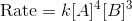If the concentration of each reactant is increased by a factor of two, how much faster will the reaction occur?

128 times faster

8 times faster

76 times faster

16 times faster

246 times faster

128 times faster

Explanation:

We can compare the rates by setting up the following equations: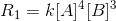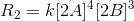We can expand the second equation by distributing the exponent: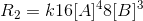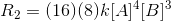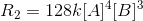We can see that the rate is multiplied by a factor of 128 when the concentration of each reactant is doubled.

### Example Question #541 : High School ChemistryIf the concentration of each reactant is increased by a factor of two, how much faster will the reaction occur?

16 times faster

8 times faster

128 times faster

246 times faster

128 times faster

Explanation:

We can compare the rates by setting up the following equations:We can expand the second equation by distributing the exponent:We can see that the rate is multiplied by a factor of 128 when the concentration of each reactant is doubled.

### Example Question #1 : KineticsIf the concentration of each reactant is increased by a factor of two, how much faster will the reaction occur?

246 times faster

8 times faster

128 times faster

16 times faster

128 times faster

Explanation:

We can compare the rates by setting up the following equations:We can expand the second equation by distributing the exponent:We can see that the rate is multiplied by a factor of 128 when the concentration of each reactant is doubled.

### Example Question #1 : Kinetics

Consider the following equation: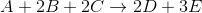The initial rate of the reaction was recorded for varying reactant concentrations and was recorded in a table: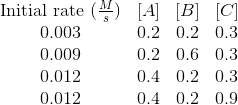Based on these experiments, what is the rate law for this reaction?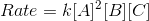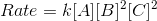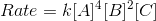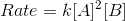Explanation:

The balanced reaction does not tell you anything about the rate law of a reaction. The rate law must be determined through experiment. The basic format of our rate law will be: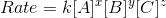In order to see how the initial concentration of a reactant affects the initial rate of the reaction, we need to find two trials where two reactants are kept constant and only one is changed. By seeing how the initial reaction rate changes, we can determine the reaction order for each particular reactant and fill in the exponent values.

When the concentration of A is doubled, the initial reaction rate is quadrupled. This can be seen by comparing trials 1 and 3. As a result, the reaction is second order with respect to A.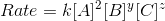When the concentration of B is tripled, the initial reaction rate is tripled. This can been seen by comparing trials 1 and 2. As a result, the reaction is first order with respect to B.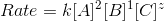Finally, the initial reaction rate will not change regardless of the concentration change of C. This can be seen by comparing trials 3 and 4. This makes the reaction zero order with respect to C.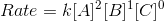### Example Question #7 : Kinetics

Consider the following reaction: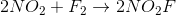The above reaction is actually composed of two steps that take place at different rates:

1.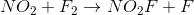(slow step)

2.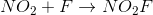(fast step)

Based on this, what is the rate law for the reaction?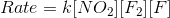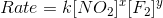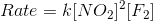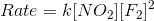Explanation:

For reactions with multiple steps, the step with the slowest rate will determine the rate law. In this reaction, the slowest step is the first step, so the reactants in that step will be the components of the rate law.

The first step is written as:Using the reactants, we can determine the rate law to beNote that we cannot determine the value of the exponents, since they will need to be found experimentally.

### Example Question #1 : Kinetics

The overall reaction can only proceed as quickly as the __________.

products are removed

intermediate is decomposed

rate-determining step